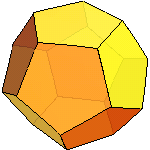t06 ⊾ 08 July 2011 ⊿ Name:

1. __________ A ball bounce function is given by $f\left(x\right)=100×{0.85}^{x}$ where x is the bounce number and f(x) is the bounce height. Evaluate this function at x = 10 bounces.
2. __________ A ball bounce function is given by $f\left(x\right)=100×{0.85}^{x}$ where x is the bounce number and f(x) is the bounce height. Solve for the bounce number x where f(x) is 8.73542 centimeters high .
3. __________ The compound interest formula is given by: $A=P{\left(1+\frac{r}{n}\right)}^{nt}$ For a principal P of \$460, an interest rate r of 0.07, compounded n = 4 times a year, calculate the Amount A after a time t of nineteen years.
4. __________ The continously compounding interest formula is given by: $A=P{e}^{rt}$ For a principal P of \$460, an interest rate r of 0.05, calculate the Amount A after a time t of ten years.
5.Transit has a growth rate given by the following table:
Age in months
post-conception
Actual mass
in kilograms
9.0 3.35
10.24.69
11.35.97
13.27.59
15.38.13
21.79.29
33.710.91

Enter the data in a LibreOffice.org Calc spreadsheet. Insert a logarithmic trend line and show the equation. Write the equation below:

6. ___________ Transit has a growth rate curve given by the equation above. Use the logarithmic equation to predict the mass of the baby at 27 months post-conception.
7. __________ Transit has a growth rate curve given by the equation above. Use the logarithmic equation to solve for the month in which the baby will have a mass of 10 kilograms.
8. The soil on Bikini atoll in the Marshall Islands is contaminated by the radioactive element Cesium-137. Like all radioactive elements, Cesium-137 will eventually decay into non-radioactive elements. The rate of decay is an exponential decay given by the formula
$N=P{e}^{\left(-0.023t\right)}$ where P is the starting amount of Cesium in the soil in kilograms, t is the number of years, and N is the remaining amount of radioactive Cesium after that number of years.

__________ If there were 100 kilograms of Cesium-137 in the soil in 1954 as a result of the Castle Bravo thermonuclear hydrogen bomb test, how many kilograms will be in the soil now in 2011?
9. __________ How many years after 1954 until there is only 1 kilogram of the original 100 kilograms of Cesium-137 left in the soil?

The circle has a radius r = 100. The shape is a seven sided heptagon. The center of the circle is (100,100). The coordinate system is SVG with y values increasing as one moves down the page. θ is theta. φ is phi.
10. ( _____ , _____ ) What are the coordinates of A, the point at the very top of the seven-sided heptagon?
11. __________ Given r = 100 and θ = 38.57°, calculate the length of B, the adjacent side.
12. ( _____ , _____ ) What are the coordinates of point C?
13. __________ Given r = 100 and θ = 38.57°, calculate the length of D, the opposite side.
14. ( _____ , _____ ) What are the coordinates of point E?
15. __________ Given r = 100 and φ = 25.71°, calculate the length of F, the adjacent side.
16. ( _____ , _____ ) What are the coordinates of point G?
17. __________ Given r = 100 and φ = 25.71°, calculate the length of H, the opposite side.
18. ( _____ , _____ ) What are the coordinates of point I?
19. A RipStik was ridden across a wet cloth towel soaked in water with food color. The RipStik was then swizzled across a large sheet of presentation paper. The swizzle wave can be seen in the diagram below.

λ = _______________ Determine the wavelength λ of the RipStik swizzle wave.
20. a = _______________ Determine the amplitude a of the RipStik swizzle wave.
21. f(x) = ______ sin( _________ x). Given the general form $f\left(x\right)=a\mathrm{sin}\left(\frac{2\pi x}{\lambda }\right)$, write the equation for the RipStik swizzle wave.
22. ________ Calculate $\mathrm{arcsin}\left(\frac{\sqrt{3}}{2}\right)$, report the result in degrees.
23. __________ For a right triangle with an opposite side of length 140 and a hypotenuse of 221, find the angle θ in degrees.
24. __________ For a right triangle with an opposite side of length 140 and a hypotenuse of 221, find the length of the adjacent side x.
25. ______, _____ Find the other two numbers in a Pythagorean triple where one of the three numbers is 28.
26. _________ The equation for a projectile is given by: $\text{distance}=\frac{2{v}^{2}\mathrm{sin}\left(\text{θ}\right)\mathrm{cos}\left(\text{θ}\right)}{\mathrm{9.8}}$
For a velocity v of 28 m/s, and an angle of 45°, calculate the distance d.
27. _________ Solve for the velocity v: $\frac{2{v}^{2}\mathrm{sin}\left(40\right)\mathrm{cos}\left(40\right)}{\mathrm{9.8}}=\mathrm{10.0491}$ with the angles given in degrees.
28. Solve: 49.4109 sin(x) cos(x) = 24.3301 for 0 < x < π/2.
Report the angle x in radians for the solution between 0 and π/2. Find the answer in decimal radians, not in arctan form. If there is more than one answer, report both. Note that π/2 is also equal to 1.57.

29. __________ For a force F of 30 Newtons, a distance of travel d of 800 meters, and an angle theta θ of 35°, use the dot product to calculate the work done pulling a toy wagon with the handle held at that angle.
30. __________ For a force F of 30 Newtons, a lever arm distance d of 0.4 meters, and an angle theta θ of 35°, use the cross product to calculate the torque on a toy wagon with the handle held at that angle.
31. Solve: (x − 5)² + (y − 4)² = 49, (x + 5)² + (y + 4)² = 81 for x and y. List all real solutions. Keep only two decimal places!

32.____________ Calculate the magnitude for the vector {696, 697}.
33. ____________ Calculate the angle relative to the horizontal axis for the vector {696, 697}.
34. ______________________ What is the name of the shape?